Finding values of expressions

Chapter 8 Class 10 Introduction to Trignometry
Concept wise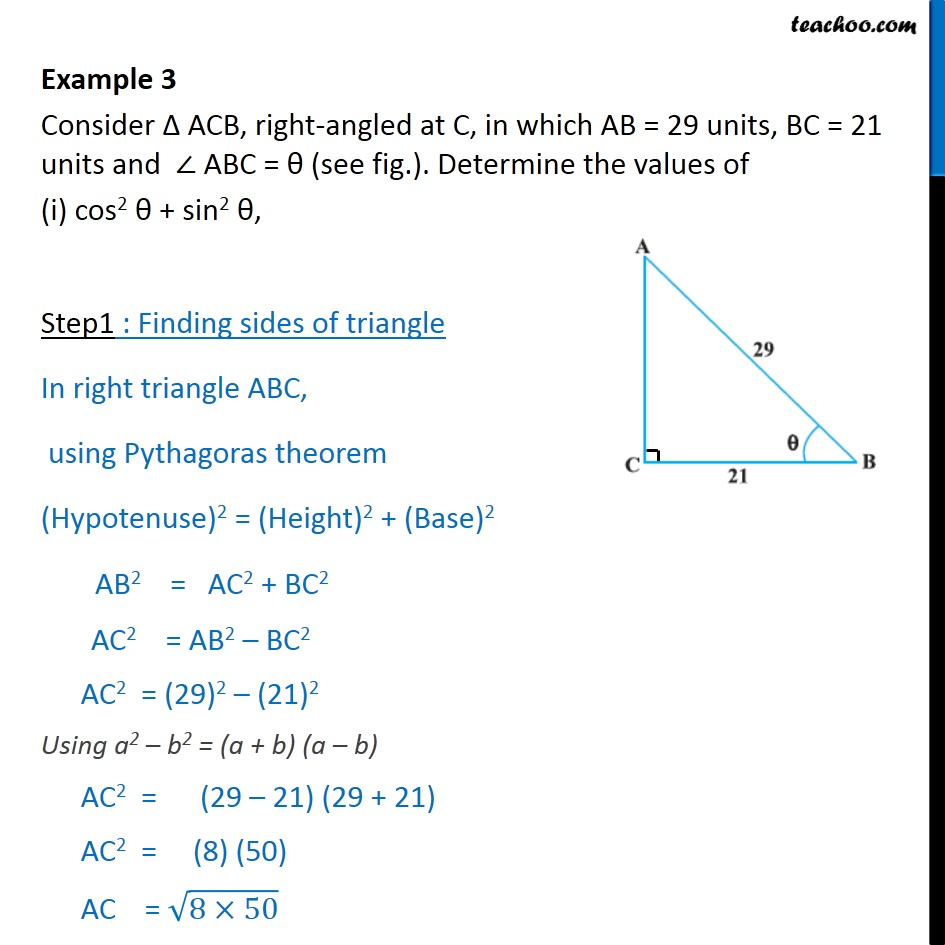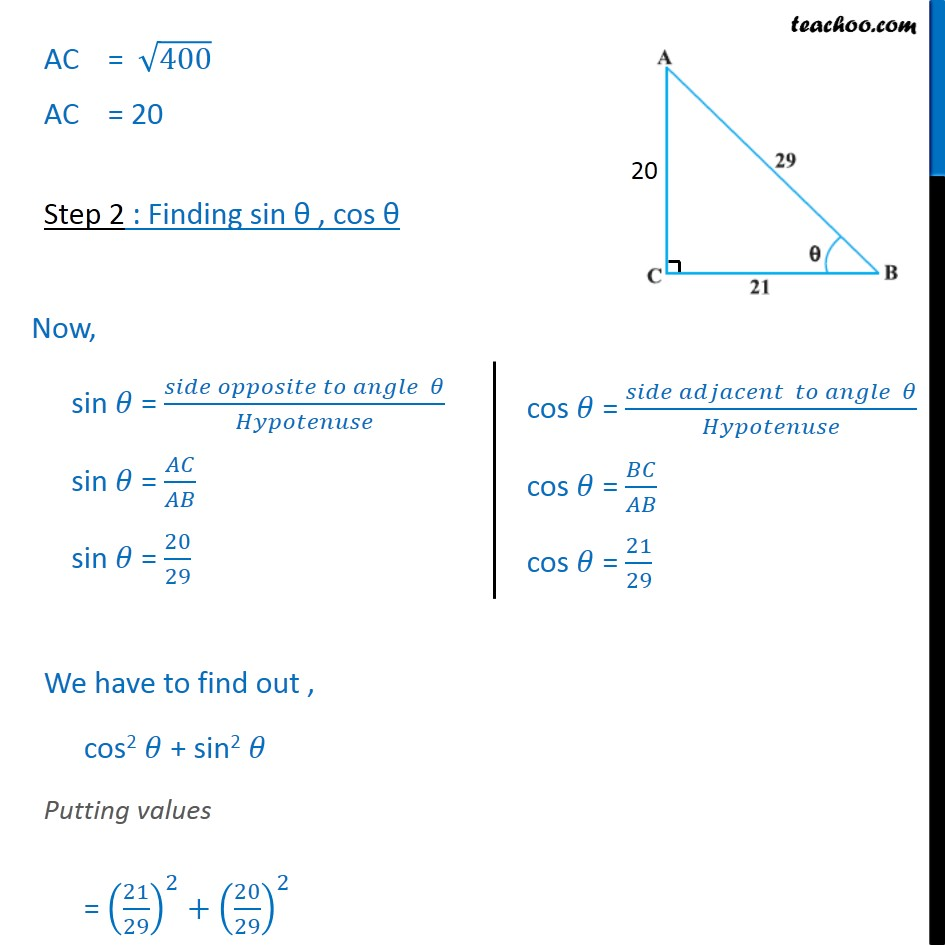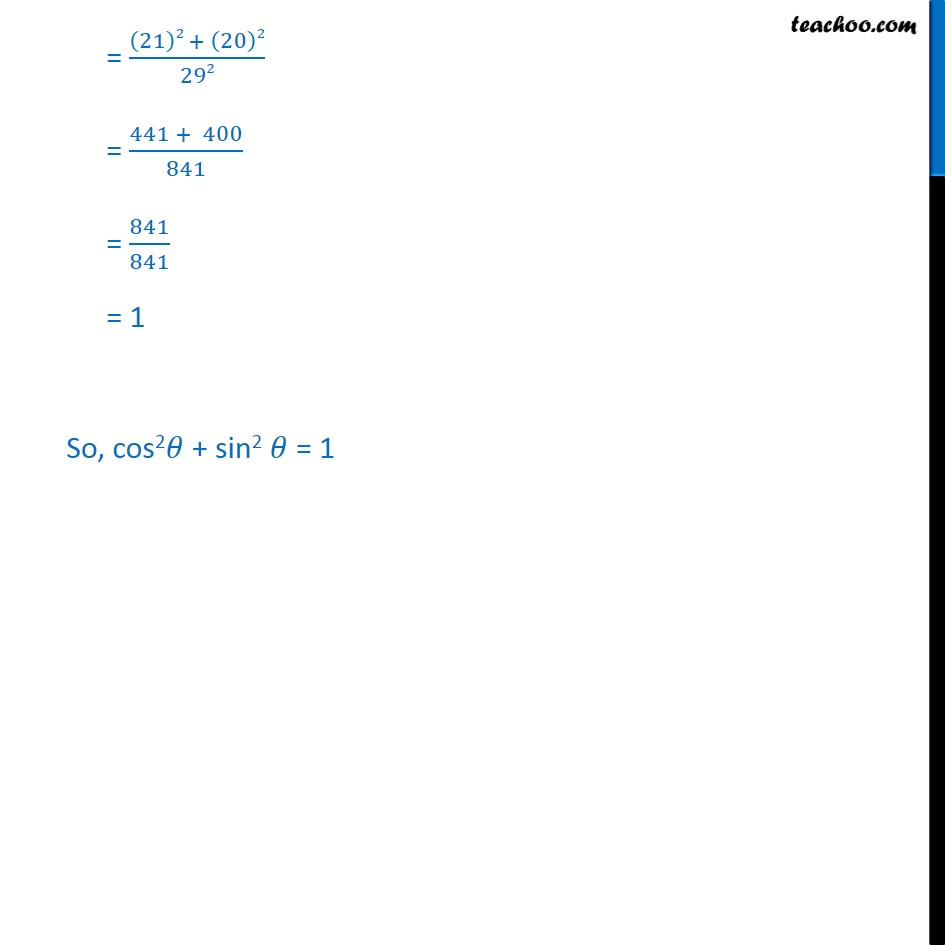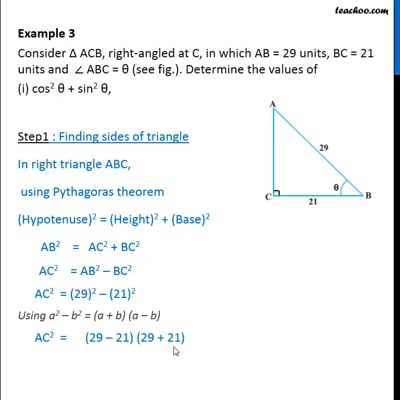This video is only available for Teachoo black users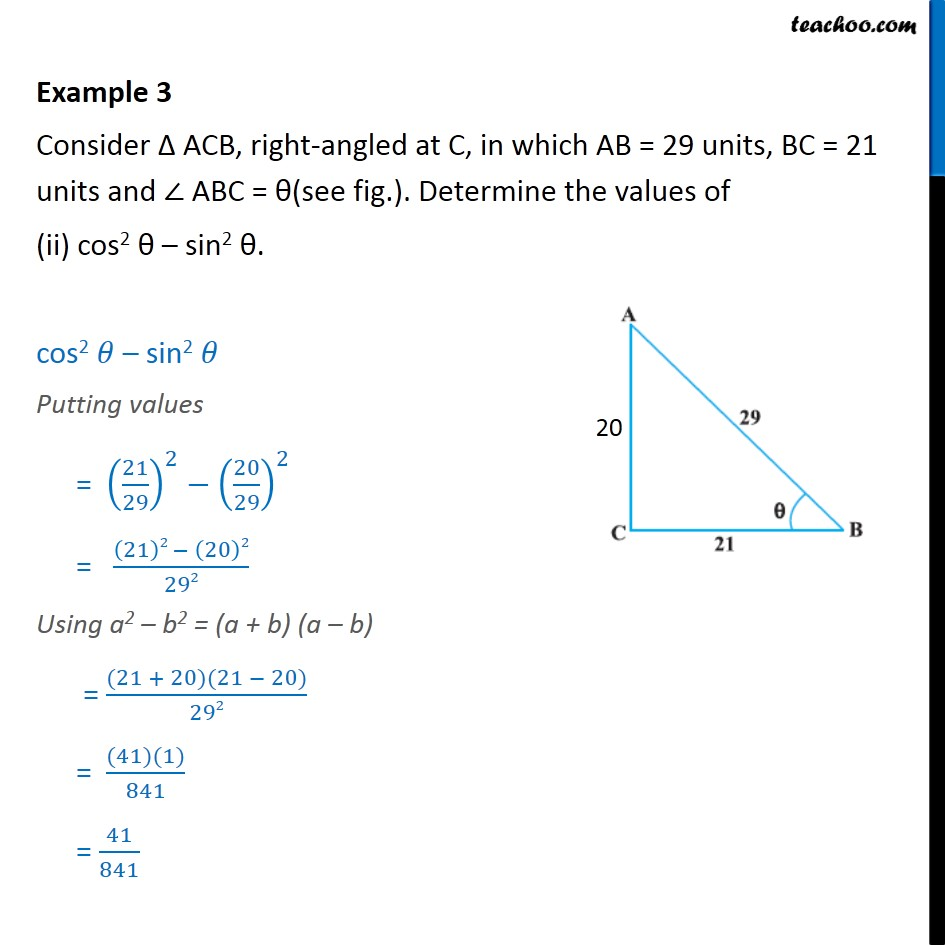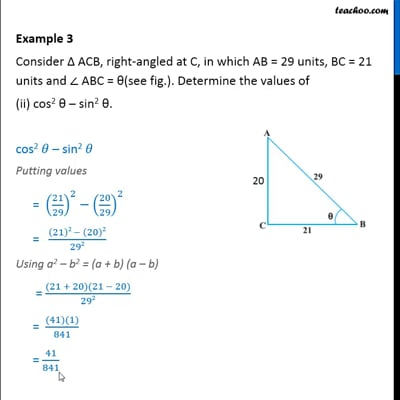This video is only available for Teachoo black users

Introducing your new favourite teacher - Teachoo Black, at only ₹83 per month

### Transcript

Example 3 Consider ACB, right-angled at C, in which AB = 29 units, BC = 21 units and ABC = (see fig.). Determine the values of (i) cos2 + sin2 , Step1 : Finding sides of triangle In right triangle ABC, using Pythagoras theorem (Hypotenuse)2 = (Height)2 + (Base)2 AB2 = AC2 + BC2 AC2 = AB2 BC2 AC2 = (29)2 (21)2 Using a2 b2 = (a + b) (a b) AC2 = (29 21) (29 + 21) AC2 = (8) (50) AC = (8 50) AC = 400 AC = 20 Step 2 : Finding sin , cos We have to find out , cos2 + sin2 Putting values = (21/29)^2+(20/29)^2 = ((21)2 + (20)2)/292 = (441 + 400)/841 = 841/841 = 1 So, cos2 + sin2 = 1 Example 3 Consider ACB, right-angled at C, in which AB = 29 units, BC = 21 units and ABC = (see fig.). Determine the values of (ii) cos2 sin2 . cos2 sin2 Putting values = (21/29)^2 (20/29)^2 = ((21)2 (20)2)/292 Using a2 b2 = (a + b) (a b) = ((21 + 20)(21 20))/292 = ((41)(1))/841 = 41/841